# Data Science : What are vectors, Maths!This is a vector: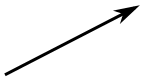A vector has a magnitude (size) and direction:The length of the line shows its magnitude and the arrowhead points in the direction.And it doesn’t matter which order we add them, we get the same result: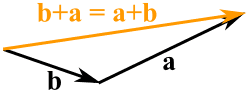### Example: A plane is flying along, pointing North, but there is a wind coming from the North-West.The two vectors (the velocity caused by the propeller, and the velocity of the wind) result in a slightly slower ground speed heading a little East of North.

If you watched the plane from the ground it would seem to be slipping sideways a little.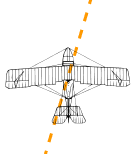Have you ever seen that happen? Maybe you have seen birds struggling against a strong wind that seems to fly sideways. Vectors help explain that.

Velocity, acceleration, force and many other things are vectors.

## Subtracting

We can also subtract one vector from another:

• first, we reverse the direction of the vector we want to subtract,
• then add them as usual: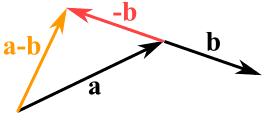a − b

## Notation

A vector is often written in bold, like a or b.

 A vector can also be written as the letters of its head and tail with an arrow above it, like this:## Calculations

Now … how do we do the calculations?

The most common way is to first break up vectors into x and y parts, like this: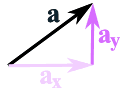The vector a is broken up into
the two vectors ax and ay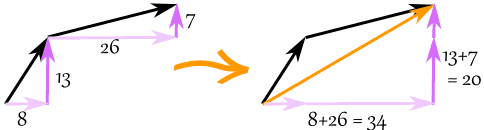The vector (8,13) and the vector (26,7) add up to the vector (34,20)

### Example: add the vectors a = (8,13) and b = (26,7)

c = a + b

c = (8,13) + (26,7) = (8+26,13+7) = (34,20)

When we break up a vector like that, each part is called a component.

## Subtracting Vectors

To subtract, first reverse the vector we want to subtract, then add.

### Example: subtract k = (4,5) from v = (12,2)

a = v + −k

a = (12,2) + −(4,5) = (12,2) + (−4,−5) = (12−4,2−5) = (8,−3)

## Magnitude of a Vector

The magnitude of a vector is shown by two vertical bars on either side of the vector:

|a|

OR it can be written with double vertical bars (so as not to confuse it with absolute value):

||a||

We use Pythagoras’ theorem to calculate it:

|a| = √( x2 + y2 )

### Example: what is the magnitude of the vector b = (6,8) ?

|b| = √( 62 + 82 ) = √( 36+64 ) = √100 = 10

A vector with magnitude 1 is called a Unit Vector.

## Vector vs Scalar

scalar has a magnitude (size) only.

Scalar: just a number (like 7 or −0.32) … definitely not a vector.

vector has magnitude and direction and is often written in bold, so we know it is not a scalar:

• so c is a vector, it has magnitude and direction
• but c is just a value, like 3 or 12.4